## use pytest.mark.parametrize to remove redundancy in your test suite

The further you go down the rabbit hole that is programming, the more you will get exposed to a thing called unit testing, or testing in general and eventually to test-driven development. There is a couple of things you need to get started. You need to understand what testing is, and why you need to test your code and you will have to face the hard reality: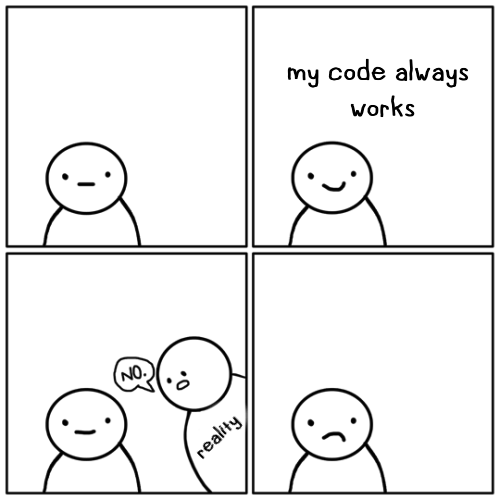After that, you will pick your test framework and get to work. I opted for pytest for no particular reason at first. I noticed it was used in other teams at work, and I found it to be mentioned a little more often at conferences than other frameworks. I got the hang on it fairly quickly, yet I’m sure to not tap into its full potential and I’m still discovering things the further I get that make testing even more efficient. One of those things is the parametrizing of test functions.

## example function

Say you have a simple function that takes two `float` or `int` values and adds them together and throws a custom `TypeError` if the values your function received are not int or float.

``````def add(a: Union[float, int], b: Union[float, int]) -> Union[float, int]:

if any([isinstance(a, _type) for _type in [int, float]]) and any(
[isinstance(b, _type) for _type in [int, float]]
):
return a + b
else:
raise TypeError("a and b can either be int or float")
``````

Now you want to test that function using pytest

``````def test_example_success():
result = add(a=2, b=3)
assert result == 5
``````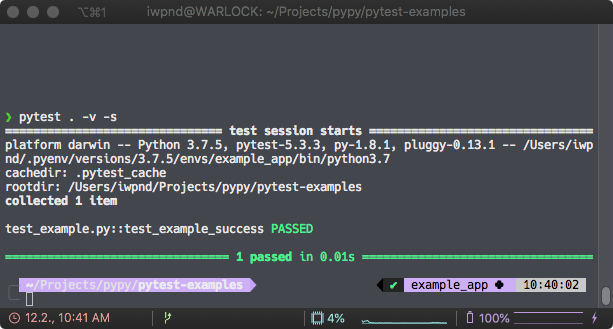and it passes. No big surprise here.

### redundancy

So what you want to do now is to check whether `TypeError` is ever raised, if the input is something other than an `int` or `float`. My naive understanding was, that I would just create a couple of test functions, that would test different types of inputs and see if `pytest.raises` would raise that `TypeError`.

``````def test_example_add_fails_string():
with pytest.raises(TypeError):
result = add(a="2", b="3")

with pytest.raises(TypeError):
result = add(a=, b=)
``````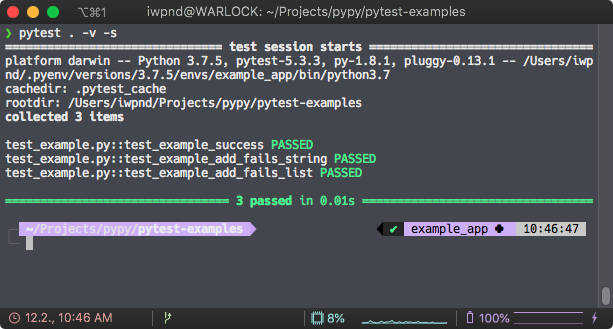While that works just fine, there is quite some redundancy in here, even if we are just testing that simple `add()` function. So to avoid that we can use the `pytest.mark.parametrize` decorator that allows us to test a multitude of arguments against the same test functions, without having to duplicate the test function itself.

### parametrizing

``````@pytest.mark.parametrize(
"a, b, expectation", # parameter for the test function
[
("3", 4, pytest.raises(TypeError)),
(3, "4", pytest.raises(TypeError)),
(["3"], ["4"], pytest.raises(TypeError)),
("3", "4", pytest.raises(TypeError)),
# [...]
]
)
def test_example_add_raises(a, b, expectation):
with expectation:
``````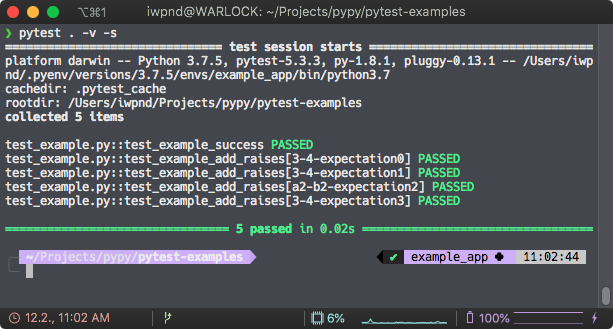As you can see pytest runs the same test function but separately with the parameters that you set in the `decorator` which essentially removes code duplication in your unit tests. And since every set of input parameters is appended in brackets in the pytest log, you can easily spot which input does not behave as intended. Of course, you can use the same idea to test the input that you want to succeed.

``````@pytest.mark.parametrize(
"a, b, expectation",
[
(1, 3, 4),
(1.3, 3.7, 5),
(-1, 4, 3)
]
)
def test_example_success(a, b, expectation):
result = add(a=a, b=b)
assert result == expectation
``````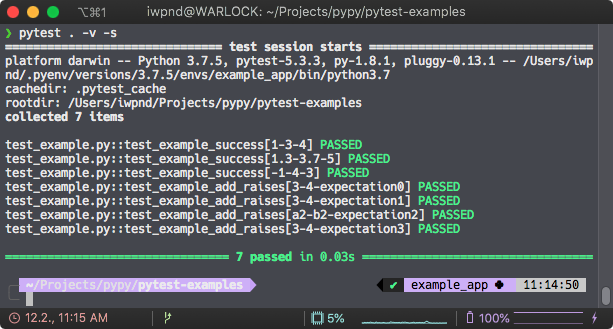That’s it for a quick introduction. There’s a lot more to learn on parametrize-basics and even more when you went past the basics.

Next up, `fixtures`.

· python, pytest